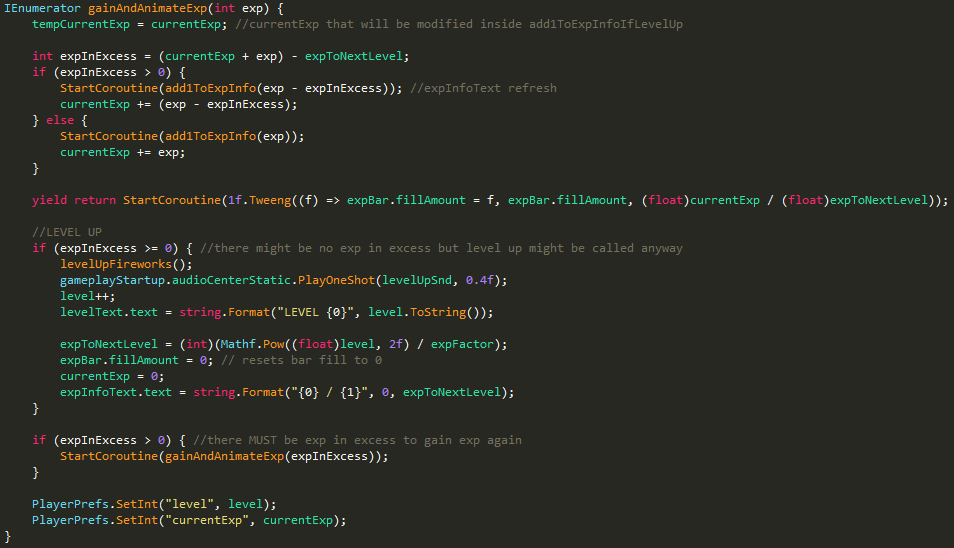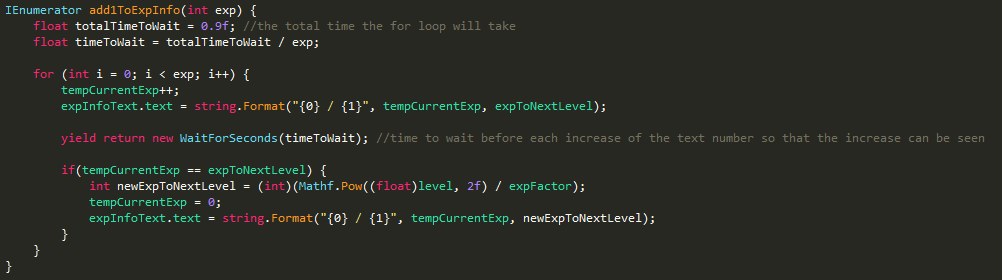### 8/22/2016In Ragdoll Slash I decided to implent an exp-based level system that will allow the user to get rewards.
I had some problems with showing the exp number increase and the bar filling when the user leveled up more than once after a single play so I came up with a recursive function that works whether you don't level up or you level up 1 or more times.In the image above you can see the gainAndAnimateExp() method which is a coroutine (thus returning IEnumerator) that is called every time there's experience to gain.

Suppose we currently have 0 exp out of 25 and get 30 exp points for completing an objective.

gainAndAnimateExp() is called once with 30 as exp parameter, here's what happens:

tempCurrentExp (an int to store the current exp we have) is set to be equal to currentExp (how much exp we have at the moment).

• we calculate how much exp we have that goes beyond our limit (that is expToNextLevel = 25 in this case) with the formula:

`​expInExcess = (currentExp + exp) - expToNextLevel; `

expInExcess = (0 + 30) - 25 = 5

Now we know we have 5 exp points in excess.

• if we have any exp in excess (expInExcess > 0) add1ToExpInfo() coroutine is started (see the image at the bottom). This is the coroutine that increases the number shown on the bar ("0 / 25" in this case). It simply has the exp we want to add passed as a parameter and a for loop that increases tempCurrentExp (the variable in which we previously stored our currentExp) by 1 for exp times. We use yield return new WaitForSeconds(timeToWait); to make the function wait for timeToWait so that we can actually see the number increasing. timeToWait is calculated as totalTimeToWait / exp so that the time the for loop will take to finish is always equal to totalTimeToWait, no matter how much exp we gain.

For example: if we gain 100 exp the for loop will wait for 0.009 seconds each cycle, for a total of 0.9 seconds. If we gain 30 exp the for loop will wait for 0.03 seconds each cycle, for a total of 0.9 seconds.

If tempCurrentExp is equal to expToNextLevel then we reached the exp needed to level up and tempCurrentExp will be reset to 0 and the new expToNextLevel will be calculated and shown.

• we add exp or (exp - expInExcess) (if there's any exp in excess) to currentExp to get our new current exp. We then use the Tweeng function to modify the fill amount of the image that shows the exp bar progress over a given time thus creating the progress animation.

is expInExcess >= 0? If so, we must level up for sure because currentExp has reached expToNextLevel. Here we do the level up stuff, including calculating the new expToNextLevel and resetting currentExp and the progress bar fill amount.

is expInExcess > 0? If there's any exp in excess gainAndAnimateExp() will cal itself, passing expInExcess as the amount of exp to gain. Here the process starts over, making us gain those 5 exp points that were over the limit. It will go on till expInExcess is less than or equal to 0.